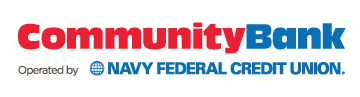NAS I Sigonella: Banking Center is now open.Enter the amount that you have available to deposit in a CD and press 'calculate' to see available terms, rates, and what your CD will be worth at maturity. A minimum deposit of \$1000 is required.

\$

This calculator is meant for estimation purposes only. Rates may change at any time and additional CD terms may apply.

## CD Calculator Results

Initial deposit amount: \$1000.00

Certificate of Deposit (CD)
Calculated Value at Maturity
Interest Rate**
Annual Percentage Yield*
Min Deposit to Open
Frequency of Compounding
Min Daily Balance
36 Months
Calculated Value at Maturity \$1,069.03
Interest Rate** 2.25%
APR* 2.25%
Min Deposit to Open \$1000
Frequency of Compounding Annually
Min Daily Balance \$1000
24-35 Months
Calculated Value at Maturity \$1,044.48
Interest Rate** 2.20%
APR* 2.20%
Min Deposit to Open \$1000
Frequency of Compounding Annually
Min Daily Balance \$1000
12-23 Months
Calculated Value at Maturity \$1,020.50
Interest Rate** 2.05%
APR* 2.05%
Min Deposit to Open \$1000
Frequency of Compounding Annually
Min Daily Balance \$1000
6-11 Months
Calculated Value at Maturity \$1,008.46
Interest Rate** 1.70%
APR* 1.70%
Min Deposit to Open \$1000
Frequency of Compounding None
Min Daily Balance \$1000
90-179 Days
Calculated Value at Maturity \$1,001.99
Interest Rate** 0.80%
APR* 0.80%
Min Deposit to Open \$1000
Frequency of Compounding None
Min Daily Balance \$1000

*After your CD is opened, the interest rate is fixed for the term. A penalty may be imposed for early withdrawal. APY does not include leap year calculations.

**Annual Percentage Yield (APY) effective Dec 6, 2019 and may change at any time without prior notice. Fees could reduce earnings on the account.